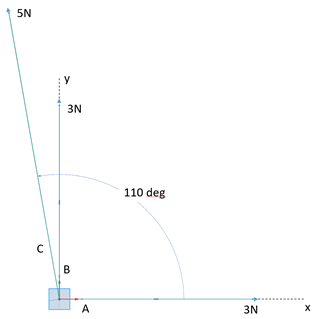## Force Vectors

Calculate the resultant magnitude from the three coplanar forces below.Hint
$$R=\sqrt{{R_{x}}^{2}+{R_{y}}^{2}}$$$Hint 2 We need to find the total forces both in the x and y directions. $$R_{x}=\sum F_{xi}$$$
$$R_{y}=\sum F_{yi}$$$$$i=A,B,C$$$
We need to find the total forces both in the x and y directions.
$$R_{x}=\sum F_{xi}$$$$$R_{y}=\sum F_{yi}$$$
$$i=A,B,C$$$$$R_{x}=3N+5cos(110)N+0N=3N-1.71N=1.29N$$$
$$R_{y}=3N+5 sin⁡(110)N+0N=3N+4.69N=7.69N$$$Finally, to solve for the magnitude: $$R=\sqrt{{R_{x}}^{2}+{R_{y}}^{2}}=\sqrt{(1.29N)^{2}+(7.69N)^{2}}=\sqrt{60.8N^{2}}=7.8N$$$
7.8N